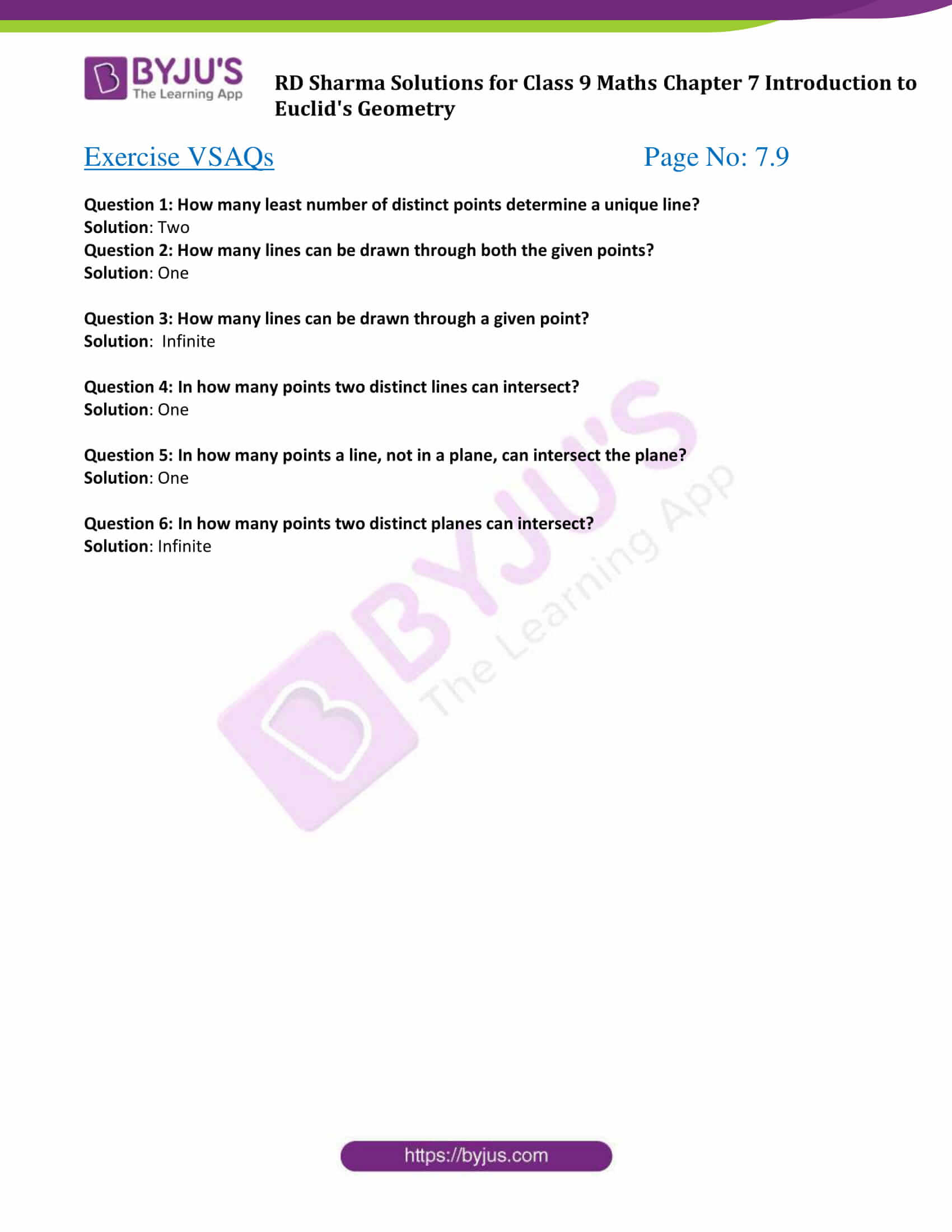# RD Sharma Solutions for Class 9 Maths Chapter 7 Introduction to Euclid's Geometry Exercise VSAQs

RD Sharma Solutions for class 9 Maths is given here for chapter 7 – Introduction to Euclid’s Geometry exercise VSAQs. Further, with the RD Sharma exercises students can understand line segment length axiom, congruent line segment length axiom and line segment construction axiom. Learn these concepts easily by practicing the questions from the exercises given in RD Sharma textbook for this chapter. Find detailed RD Sharma Solutions for Class 9 Maths Chapter 7 below.

## Download PDF of RD Sharma Solutions for Class 9 Maths Chapter 7 Introduction to Euclid’s Geometry Exercise VSAQs### Exercise VSAQs Page No: 7.9

Question 1: How many least number of distinct points determine a unique line?

Solution: Two

Question 2: How many lines can be drawn through both the given points?

Solution: One

Question 3: How many lines can be drawn through a given point?

Solution: Infinite

Question 4: In how many points two distinct lines can intersect?

Solution: One

Question 5: In how many points a line, not in a plane, can intersect the plane?

Solution: One

Question 6: In how many points two distinct planes can intersect?

Solution: Infinite

## RD Sharma Solutions for Class 9 Maths Chapter 7 Introduction to Euclid’s Geometry Exercise VSAQs

RD Sharma Solutions Class 9 Maths Chapter 7 Introduction to Euclid’s Geometry Exercise VSAQs is based on line segment length axiom, congruent line segment length axiom and line segment construction axiom. Students can go through the RD Sharma solutions for class 9 chapter 7 which have been prepared by our experts.